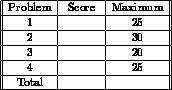Next: About this document Up: My Home Page

SUNY at Stony BrookMidterm 1
CSE 214 - Data Structures October 10, 1997

Midterm Exam

Name: Signature:
ID #: Section #:

INSTRUCTIONS:

• You may use either pen or pencil.
• Check to see that you have 4 exam pages plus this cover (5 total).
• Look over all problems before starting work.
• Your signature above signs the CSE 214 Honor Pledge: ``On my honor as a student I have neither given nor received aid on this exam.''
• Think before you write.
• Good luck!!1) (25 points) Assume that you have the linked structure on the left, where each node contains a .next field consisting of a pointer, and the pointer p points to the structure as shown. Describe the sequence of Modula-3 pointer manipulations necessary to convert it to the linked structure on the right. You may not change any of the .info fields, but you may use temporary pointers tmp1, tmp2, and tmp3 if you wish.

Many different solutions were possible, including:

```tmp1 := p;
p := p.next;
p^.next^.next := tmp1;```

2) (30 points) Write a procedure which ``compresses'' a linked list by deleting consecutive copies of the same character from it. For example, the list (A,B,B,C,A,A,A,C,A) should be compressed to (A,B,C,A,C,A). Thus the same character can appear more than once in the compressed list, only not successively. Your procedure must have one argument as defined below, a VAR parameter head pointing to the front of the linked list. Each node in the list has .info and .next fields.

`PROCEDURE compress(VAR head : pointer);`

Many different solutions are possible, but recursive solutions are particularly clean and elegant.

```PROCEDURE compress(VAR head : pointer);
VAR
second : pointer;	(* pointer to next element *)

BEGIN
IF (head # NIL) THEN
second := head^.next;
IF (second # NIL)
IF (head^.info = second^.info) THEN
head^.next = second^.next;
compress(head);
ELSE
compress(head^.next);
END;
END;
END;
END;```

3) (20 points) Provide the output of the following program:

```MODULE strange; EXPORTS main;

IMPORT SIO;

TYPE
ptr_to_integer =  REF INTEGER;
VAR
a, b : ptr_to_integer;

PROCEDURE modify(x : ptr_to_integer; VAR y : ptr_to_integer);
begin
x^ := 3;
SIO.PutInt(a^);
SIO.PutInt(x^); SIO.Nl();
y^ := 4;
SIO.PutInt(b^);
SIO.PutInt(y^); SIO.Nl();
end;

begin
a := NEW(INTEGER); b := NEW(INTEGER);
a^ := 1;
b^ := 2;
SIO.PutInt(a^);
SIO.PutInt(b^); SIO.Nl();
modify(a,b);
SIO.PutInt(a^);
SIO.PutInt(b^); SIO.Nl();

end.```

Answers:

```1 2
3 3
4 4
3 4```

4) (25 points)

Write brief essays answering the following questions. Your answer must fit completely in the space allowed

(a) Explain the difference between objects and modules? ANSWER: Several answers possible, but the basic differences are (1) the notation to use them, and (2) that objects encapsulate both procedures and data where modules are procedure oriented. (b) What is garbage collection? ANSWER: The automatic reuse of dynamic memory which, because of pointer dereferencing, is no longer accessible. (c) What might be an advantage of a doubly-linked list over a singly-linked list for certain applications? ANSWER: Additional flexibility in moving both forward and in reverse on a linked list. Specific advantages include being able to delete a node from a list given just a pointer to the node, and efficiently implementing double-ended queues (supporing push, pop, enqueue, and dequeue).Next: About this document Up: My Home Page

Steve Skiena
Thu Oct 16 17:26:17 EDT 1997